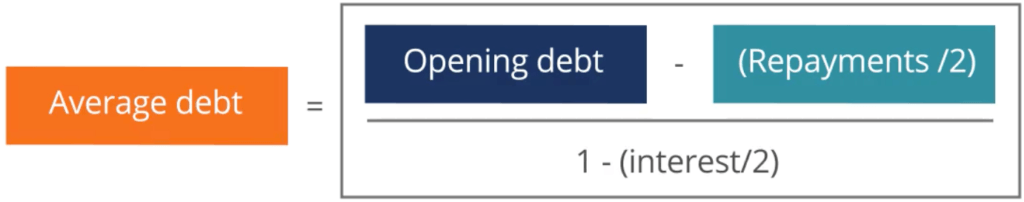# Forecasting Finance (Equity, Debt, Interest)

Part three of the four-step financial forecasting model in Excel

## Forecasting Finance: Equity, Debt and Interest

This article on forecasting finance is part three of the four-step financial forecasting model in Excel and focus on how to model equity, debt and interest. Having completed revenue forecasts down to EBIT for the income statement and operating assets forecast for the balance sheet, we can now move on to complete the balance sheet and income statement by forecasting debt and equity financing.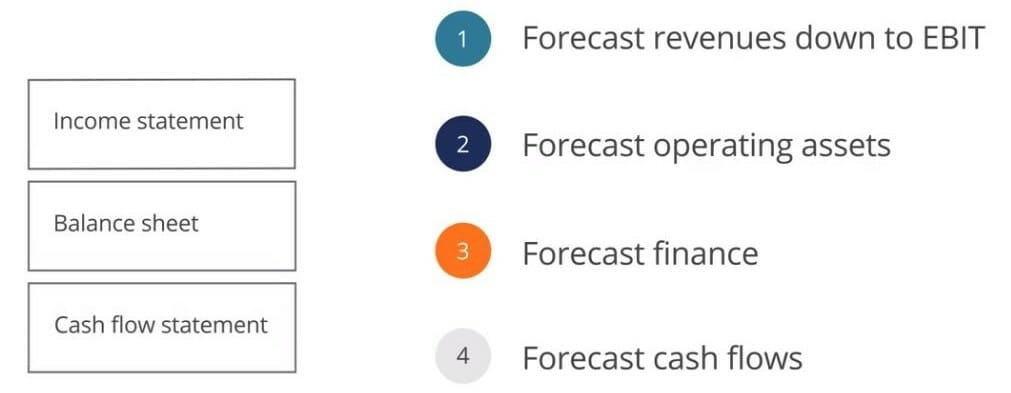• Forecast debt financing and related interest costs
• Forecast equity financing and dividends
• Use both iterative and analytical approaches to manage circular references

### Forecasting Financial Statements

Forecasting the capital structure impacts both the balance sheet and the income statement through different items, including dividends and interest expense. Forecasting equity requires forecasting stock issuance and repurchases, as well as changes in retained earnings. Changes in retained earnings will be strongly driven by forecasted net income, and dividends. When forecasted retained earnings changes in a model, users should complete a retained earnings supporting schedule section, much like how we used a note to forecast PP&E in forecasting balance sheet items.

Forecasting debt requires forecasting both short-term and long-term debt, as well as the associated interest costs. Once we’ve completed the financing forecast, we can complete the cash section, thereby completing the balance sheet. In short, cash is determined simply as the balancing figure in the balance sheet.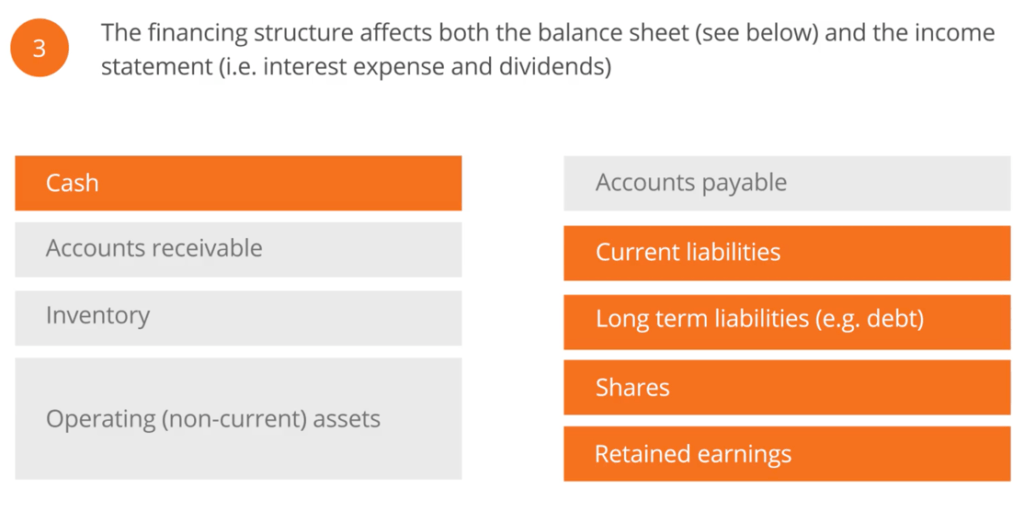### Forecasting the Capital Structure

The first question to ask when forecasting the financing structure is: what should be the split between equity and debt financing?

To answer this question, we need to consider leverage ratios, such as debt to equity, and coverage ratios, such as EBIT to interest expense.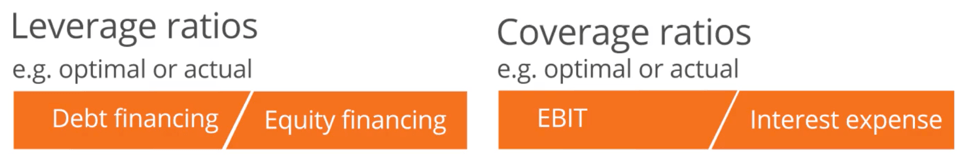Typically, financial covenants on loan agreements will dictate the maximum leverage ratios and minimum coverage ratios that can be factored into our model. However, if the leverage or coverage information is not available, we can at least consider management’s willingness to take on additional debt or equity. Moreover, we can use the company’s current level of access to the debt and equity capital markets.

### The Practicalities of Forecasting Finance

There are many practical modeling issues that need to be considered in forecasting finance, particularly in forecasting the capital structure, including equity, debt and interest.

A useful question to ask at the beginning is: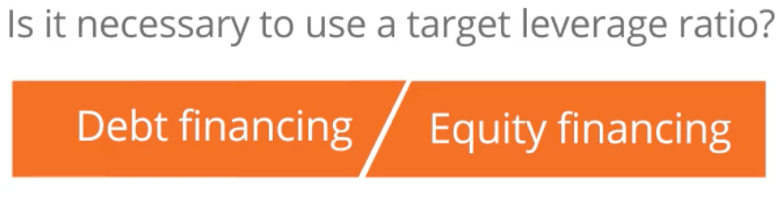In other words, do you need to forecast debt levels as a multiple of equity? If you don’t need to forecast debt levels as a multiple of equity, consider using opening debt to calculate interest and assume that long-term liabilities are constant. This will keep your model simple and straightforward. More importantly, it will minimize the chance of circular references. There are many practical modeling issues that need to be considered when forecasting the capital structure.

If you need to use target leverage figures in your model, the next question to ask is: What level of precision is required in modeling the capital structure? In the case that you need to model debt and equity issuance from first principles approach with high complexity, you will inevitably generate circular references that need to be solved. Solutions for circular references will be discussed later in this article.

The halfway point is to build your model with an intermediate level of complexity, where leverage ratios are used to calculate debt, whereas opening debt is used to calculate interest.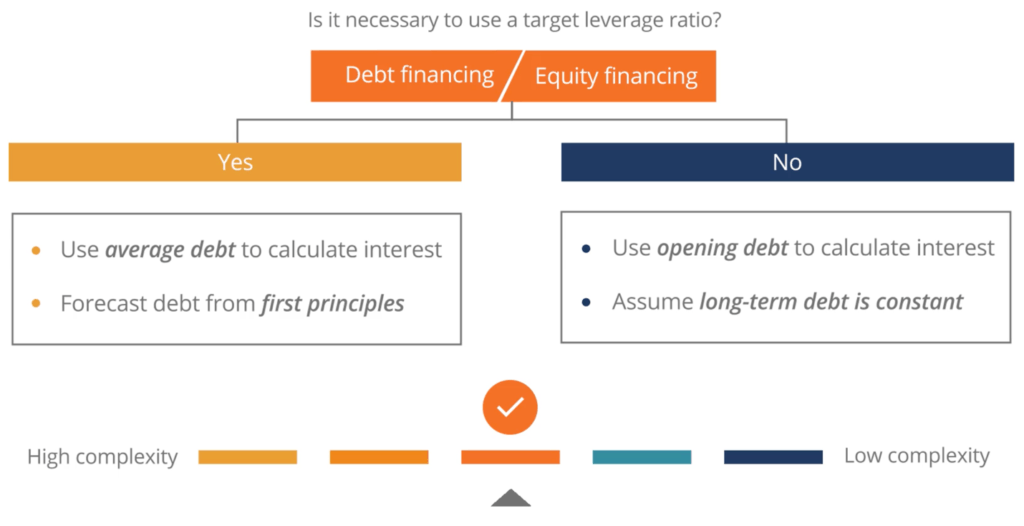### Circular References

When calculating the debt and interest expense in our financial forecast, we can either use the opening debt (which is equivalent to last year’s closing debt), this year’s closing debt, or an average of opening and closing debts. Using the average debt will typically provide the most accurate interest expense; however, it can also lead to circular references.

### Understanding the Problem: Average Debt Circular References

For simplicity’s sake, consider a debt facility (loan) with no principal repayments and where accrued interest is added to the existing principal, instead of being paid out each period. In order to get closing debt, we need two figures: opening debt and the accrued interest expense. To calculate accrued interest expense, we need two more figures: the interest rate and average debt. However, in order to calculate average debt, we need both opening and closing debt. In essence, we need closing debt to calculate closing debt. This problem is an example of a circular reference.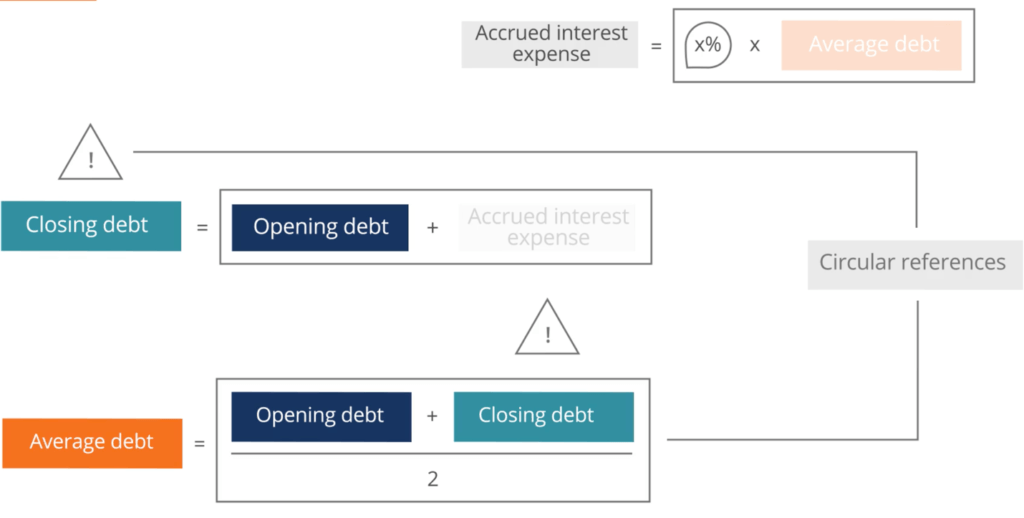### Solving Circular References with Iteration

Iteration is trying different values for model variables to ultimately find a solution. Each repetition process is called an iteration, and the result of one iteration is used as the starting point for the next iteration. For PC users in Excel 2007 and later versions, iteration is accessible on the “File” menu or Office button, then by selecting “Options”, and then by selecting “Formulas”. Check the “Enable Iteration Calculation” box.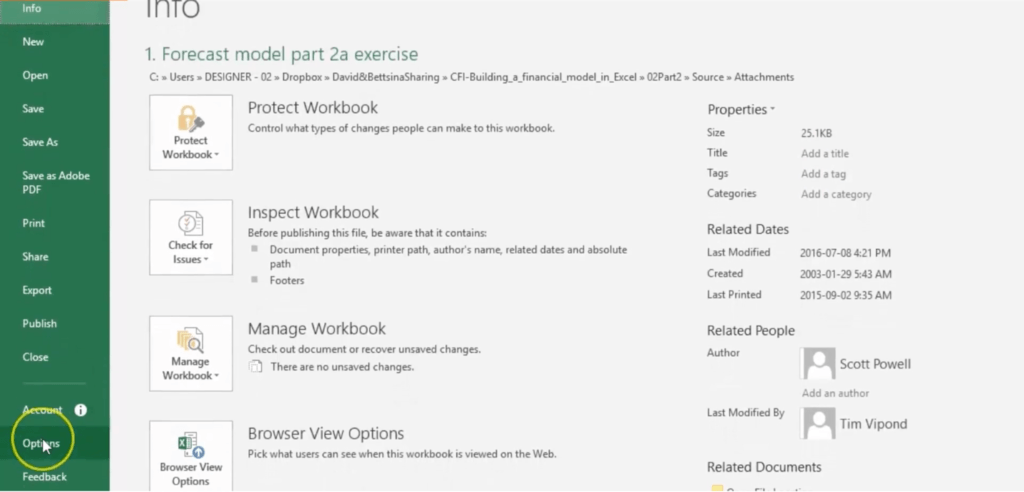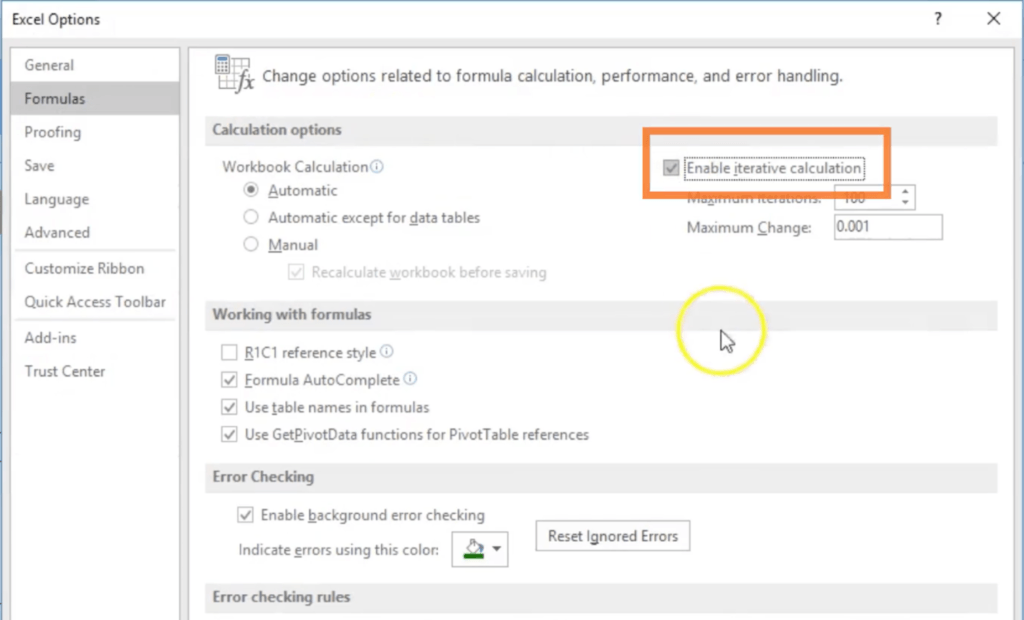For Mac users, iterative calculative can be enabled by going to the application menu bar, Excel -> Preferences -> Calculation, then check the box for “Use iterative calculation”.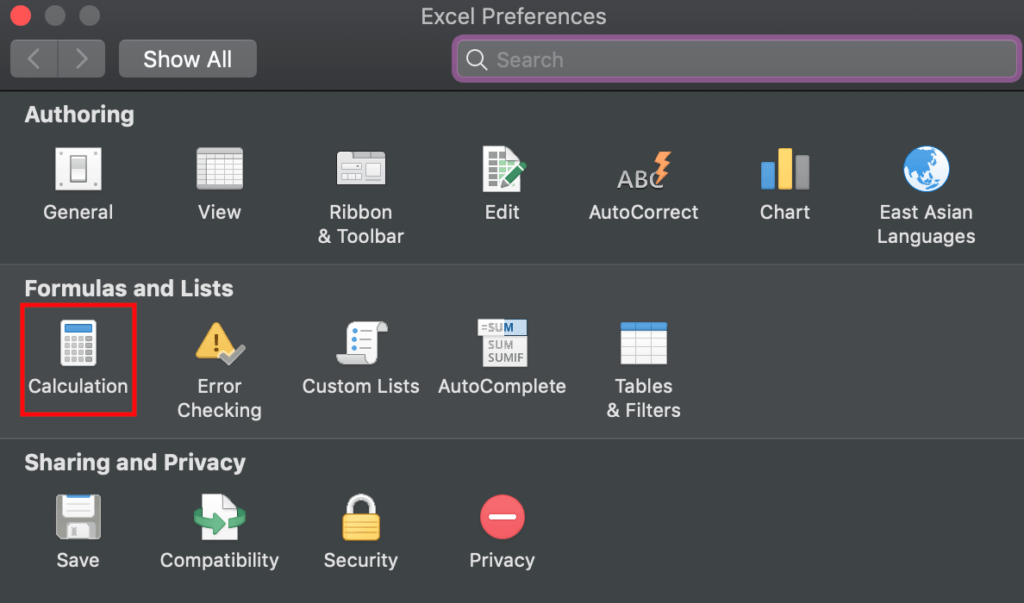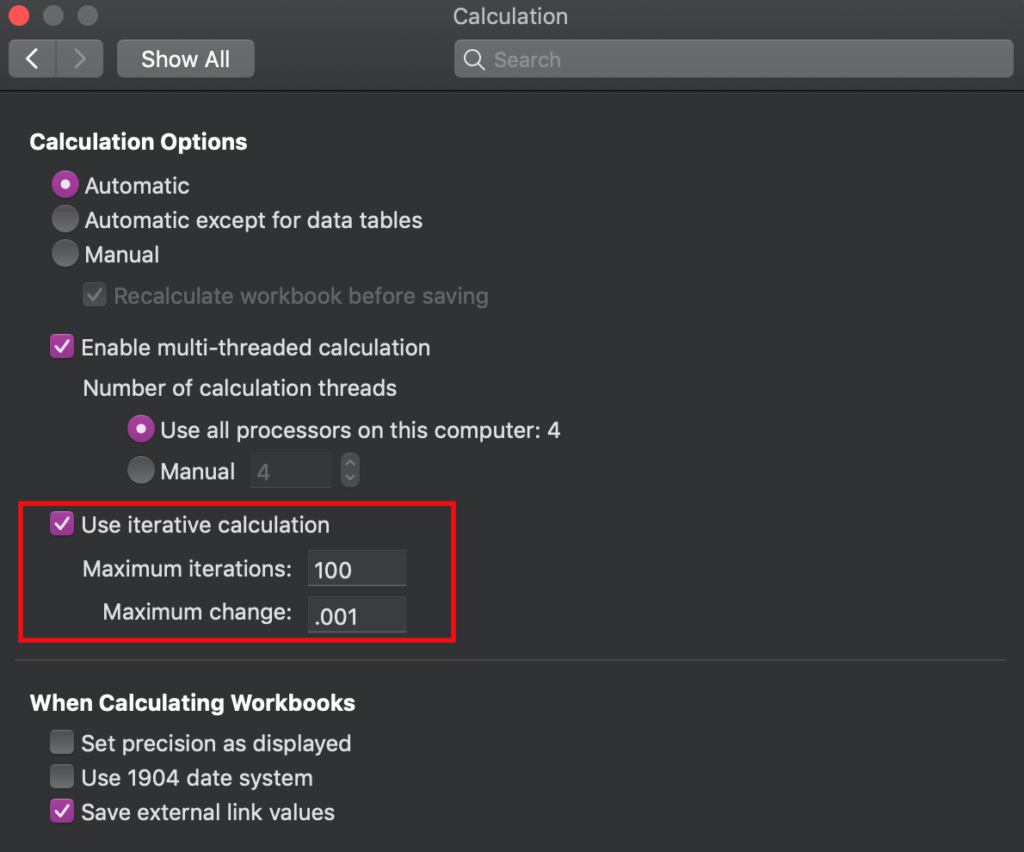### Circular References: Analytical Approach

Although the Excel function allows us to solve circular references, it must also be used with caution as Excel no longer alerts users to any additional circular references. Instead, Excel will automatically attempt to solve these circular references, which is particularly dangerous if there are multiple solutions to a circular reference.

The equation below offers an analytical solution that can be used when modeling amortizing debt that allows us to avoid from turning on the iteration function.